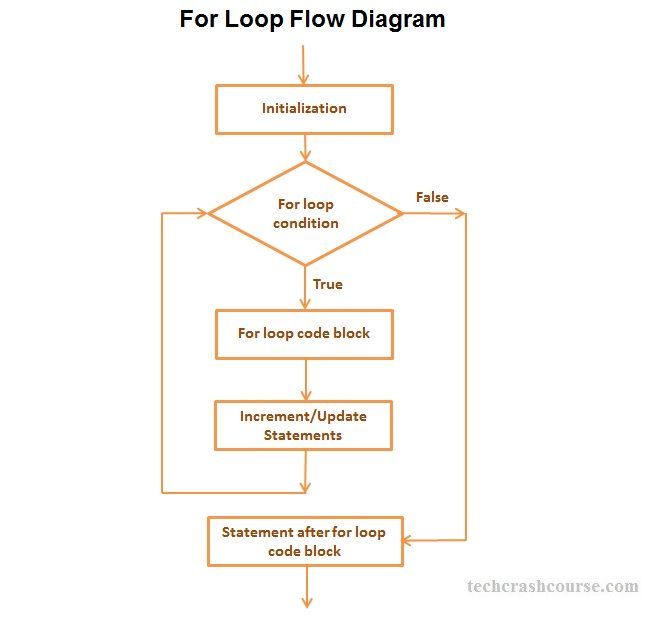# For Loop in C Programming

The for loop in C programming language is used to execute a code block several times until the given condition is true. The for loop is mainly used to perform repetitive tasks.

## Syntax of for Loop

for(initialization; condition; update) {
/* code to be executed */
}
For Example
```for(i = 1; i <= 100; i++){
printf("%d", i);
}
```
Above for loop prints integers from 1 to 100;

## For Loop Syntax Description

• Initialization : The Initialization statement allows us to declare and initialize any loop variables. It is executed only once at the beginning of the for loop.

• Condition : After initialization, condition expression is evaluated. It is a boolean expression which decides whether to go inside the loop and iterate or terminate for loop. if condition expression evaluates to true, the code block inside for loop gets executed otherwise it will terminate for loop and control goes to the next statement after the for loop.

• Update : After code block of for loop gets executed, control comes back to update statements. It allow us to modify any loop variable for next iteration of loop.

Control flow of for Loop Statement in C1. The Initialization statement will be executed first. We can declare and initialize any number of loop control variables here.

2. After the Initialization statement the condition expression is evaluated. If the value of condition expression is true then code block of for loop will be executed otherwise the loop will be terminated.

3. After execution of the code block of for loop control goes to update statements of the loop statement which modifies the loop control variables.

4. After modifying control variables in update statements, control again goes to condition expression.

5. For loop iteration continues unless condition expression becomes false or for loop gets terminated using break statement.

## C Program to show use of for Loop

```#include<stdio.h>
#include<conio.h>

int main(){
int N, counter, sum=0;
printf("Enter a positive number\n");
scanf("%d", &N);
/* Using for loop to find the sum
of all integers from 1 to N */
for(counter=1; counter <= N; counter++){
sum+= counter;
}

printf("Sum of Integers from 1 to %d = %d", N, sum);

getch();
return(0);
}
```
Above program finds sum of all integers from 1 to N, using for loop.

Output
```Enter a positive number
7
Sum of Integers from 1 to 7 = 28
```
For Loop Facts
• The condition_expression in for loop is a boolean expression. It must evaluate to true or false value(In C, all non-zero and non-null values are considered as true and zero and null value is considered as false).

• Initialization statement, Condition expression and Update statement are all optional in for loop.

• You can use multiple initialization statements and update statements inside for loop.
For Example:
for(i=0, j=50; i < 100; i++, j--)

• Opening and Closing braces are not required for single statement inside for loop code block.
For Example:
for(i = 0; i < 100; i++)
sum+= i;

• We can also use infinite for loops like for(;;), which will never terminate. You should add terminating condition using break statement in order to terminate infinite for loop.
For Example:
for(;;){
if(.....){
break;
}
}

### C Programs using For Loop

C Program to Print All Prime Numbers between 1 to N
C Program to Find All Factors of a Number
C Program to Find Sum of All Odd Numbers Between 1 to N
C Program to Find Sum of All Even Numbers Between 1 to N
C Program to Print Odd Numbers Between 1 to 100
C Program to Print Even Numbers Between 1 to 100
C Program to Print Natural Numbers in Reverse Order from 1 to N
C Program to Print Natural Numbers from 1 to N